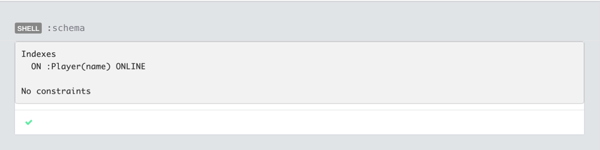# Neo4j 2.0: Labels, indexes and the like

Last week I did a couple of talks about modelling with Neo4j meet ups in Amsterdam and Antwerp and there were a few questions about how indexing works with labels that are being introduced in Neo4j 2.0

As well as defining properties on nodes we can also assign them a label which can be used to categorise different groups of nodes.

For example in the football graph we might choose to tag player nodes with the label 'Player':

``````
CREATE (randomPlayer:Player {name: "Random Player"})
``````

If we then wanted to find that player we could use the following query:

``````
MATCH (p:Player)
WHERE p.name = "Random Player"
RETURN p
``````

A common assumption amongst the attendees was that labelled nodes are automatically indexed but this isn't actually the case which we can see by profiling the above query:

``````
\$ PROFILE MATCH (p:Player) WHERE p.name = "Random Player" RETURN p;
==> +-----------------------------------+
==> | p                                 |
==> +-----------------------------------+
==> | Node{name:"Random Player"} |
==> +-----------------------------------+
==> 1 row
==>
==> Filter(pred="(Product(p,name(0),true) == Literal(Random Player) AND hasLabel(p:Player(8)))", _rows=1, _db_hits=524)
==> NodeByLabel(label="Player", identifier="p", _rows=524, _db_hits=0)
``````

Instead what we have is a 'label scan' whereby we search across the nodes labelled as 'Player' check whether they have a property 'name' which matches 'Random Player' and then return them if they do.

This is different than doing a 'full node scan', checking for the appropriate label and then property. e.g.

``````
\$ PROFILE MATCH p WHERE "Player" IN LABELS(p) AND p.name = "Random Player" RETURN p;
==> +-----------------------------------+
==> | p                                 |
==> +-----------------------------------+
==> | Node{name:"Random Player"} |
==> +-----------------------------------+
==> 1 row
==>
==> Filter(pred="(any(-_-INNER-_- in LabelsFunction(p) where Literal(Player) == -_-INNER-_-) AND Product(p,name(0),true) == Literal(Random Player))", _rows=1, _db_hits=524)
==> AllNodes(identifier="p", _rows=11443, _db_hits=11443)
``````

If we want to index a specific property of 'Player' nodes then need to explicitly index that property for that label:

``````
\$ CREATE INDEX ON :Player(name);
==> +-------------------+
==> | No data returned. |
==> +-------------------+
==> 0 ms
``````

If we want to see the indexes defined on our database we can run the following command in webadmin:

``````
\$ schema
==> Indexes
==>   ON :Player(name) ONLINE
==>
==> No constraints
``````

or its equivalent in Neo4j browser:Now if we repeat our initial query we can see that it's a straight schema/index lookup:

``````
\$ PROFILE MATCH (p:Player) WHERE p.name = "Random Player" RETURN p;
==> +-----------------------------------+
==> | p                                 |
==> +-----------------------------------+
==> | Node{name:"Random Player"} |
==> +-----------------------------------+
==> 1 row
==>
==> SchemaIndex(identifier="p", _db_hits=0, _rows=1, label="Player", query="Literal(Random Player)", property="name")
``````

Based on a few runs of the query with and without the index defined it takes 1ms and 10ms respectively. The 'full node scan' approach takes ~ 40ms and that's with a very small database of 30,000 nodes. I wouldn't recommend it with a production load.